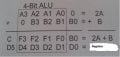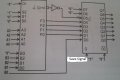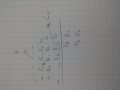# How can I do 2A+3B(3 bits) with 4 bit ALU?

#### ulas94

Joined Mar 26, 2016
7
And later (F=2A+3B) save it 8-bit register.

#### ScottWang

Joined Aug 23, 2012
7,326
Go to study 74HC181 then you will get the answer.

#### ulas94

Joined Mar 26, 2016
7
For example I have two variable A and B which are 4-bit and I want to make 2A plus B and save the answer in 8-bit register.

Solution:Answer of my question will be like this but I can't do 2A plus 3B ( A and B 3-bits) and I need help#### ScottWang

Joined Aug 23, 2012
7,326
Is this homework or personal learning?

#### ulas94

Joined Mar 26, 2016
7
It is a question which has potatial to be in the exam#### WBahn

Joined Mar 31, 2012
28,171
What is the maximum value that 2A + 3B can have? Can that be represented by the output, including carry, of a 4-bit adder?

How many bits are required to represent it?

You circuit diagram embodies some of what you need to do. Do you know which parts those are? Did you come up with that circuit diagram yourself? If so, think about why you made the connects you did and what parts of the math are taken care of and what parts aren't.

And remember that it IS a math function you are trying to implement, so play with the math.

For example, see if something like

Y = 2A + 3B = 2(A+B) + B

helps you out.

#### kubeek

Joined Sep 20, 2005
5,776
I think you should be able to use the S inputs, so that you can put A<<1 to Ain, B<<1 to Bin and B to Sin so that you get 2A+2B+1B.
(A<<1 being A shifted left by one bit)

Last edited:

#### ulas94

Joined Mar 26, 2016
7
I think put B to Select pins wrong choice because if I apply 000 to B , the F will be 0 although A is not 0

#### kubeek

Joined Sep 20, 2005
5,776
Oh sorry my mistake, i thought that S was the carry in, when in fact it is the mode select. Disregard my previous post.
My first idea was that +3B could be equal to -1B if the size of the processing busses was just right. I think this idea needs some practice becaues the ALU you described doesn't really cover all the possibilities of 4bit inputs and the according results.
So i suggest you try the A-B op code and see if it gets you anywhere.

#### ulas94

Joined Mar 26, 2016
7
Yes this ALU works unsigned numbers I understand(but I don't know) so inputs must be positive therefore signed numbers can be confusing in this scenario.
My approximation like thisB0 is going to D0 directly and A0 and B0 are going to ALU's A0 and B0 respectively and B1 is going to Cn but I have hanged out there because A1+B1+B2 does not happen.Probably I should use ALU's A3 and B3 bits which are not used in the above event

#### kubeek

Joined Sep 20, 2005
5,776
can you use external gates as well, or only the ALU?

#### djsfantasi

Joined Apr 11, 2010
8,783
Is "Not Possible" a valid answer? Given that the ALU can only be used once, that would be my answer. Because the function as defined has three operations. 2A can be calculated without using the ALU. But 3B needs to use the ALU. Leaving no way (since the ALU can only be used once) to add the two terms.

#### ulas94

Joined Mar 26, 2016
7
Unfortinately only one ALU can be used#### WBahn

Joined Mar 31, 2012
28,171
Is "Not Possible" a valid answer? Given that the ALU can only be used once, that would be my answer. Because the function as defined has three operations. 2A can be calculated without using the ALU. But 3B needs to use the ALU. Leaving no way (since the ALU can only be used once) to add the two terms.
I'm not quite convinced it's not possible yet. There is an easy way to get all but the msb of B of the odd term taken care of (so 2A + 2B + B%4). But I'm not seeing any way to get that last bit in there.